# Raycasting III: Sprites

Back to index

## Introduction

The articles Raycasting and Raycasting II described how to make textured walls and floors, but something is missing. In a game, a world with only walls and floors is empty, for a game to work, there have to be goodies, enemies, objects like barrels or trees, ... These can't be drawn as wall or floor, and, in the time when raycasting games were made, can't be drawn as 3D models either. Instead, they used sprites, 2D pictures always facing to you (so they're easy to draw and require a single picture), but that become smaller if they're further away.

## How it Works

The technique used to draw the sprites is totally different from the raycasting technique. Instead, it works very similar to how sprites are drawn in a 3D engine with projections, like in the "Points, Sprites and Moving" article. Only, we have to do the projection only in 2D, and some extra techniques to combine it with raycasting are used.

Drawing the sprites is done after the walls and floor are already drawn. Here are the steps used to draw the sprites:
• 1: While raycasting the walls, store the perpendicular distance of each vertical stripe in a 1D ZBuffer
• 2: Calculate the distance of each sprite to the player
• 3: Use this distance to sort the sprites, from furthest away to closest to the camera
• 4: Project the sprite on the camera plane (in 2D): subtract the player position from the sprite position, then multiply the result with the inverse of the 2x2 camera matrix
• 5: Calculate the size of the sprite on the screen (both in x and y direction) by using the perpendicular distance
• 6: Draw the sprites vertical stripe by vertical stripe, don't draw the vertical stripe if the distance is further away than the 1D ZBuffer of the walls of the current stripe
• 7: Draw the vertical stripe pixel by pixel, make sure there's an invisible color or all sprites would be rectangles
You don't have to update the ZBuffer while drawing the stripes: since they're sorted, the ones closer to you will be drawn last, so they're drawn over the further away ones.

How to project the sprite on screen is explained in full 3D rendering mathematics (with 3D matrices and camera), for true 3D rasterizer or raytracer 3D engines, and is not explained here in the raycaster tutorial. However, here it's done in 2D and no fancy camera class is used. To bring the sprite's coordinates to camera space, first subtract the player's position from the sprite's position, then you have the position of the sprite relative to the player. Then it has to be rotated so that the direction is relative to the player. The camera can also be skewed and has a certain size, so it isn't really a rotation, but a transformation. The transformation is done by multiplying the relative position of the sprite with the inverse of the camera matrix. The camera matrix is in our case
```[planeX   dirX]
[planeY   dirY]
```
And the inverse of a 2x2 matrix is very easy to calculate
```____________1___________    [dirY      -dirX]
(planeX*dirY-dirX*planeY) * [-planeY  planeX]
```

Then you get the X and Y coordinate of the sprite in camera space, where Y is the depth inside the screen (in a true 3D engine, Z is the depth). To project it on screen, divide X through the depth, and then translate and scale it so that it's in pixel coordinates.

To place the objects in the level, many things can be done. Each object can have its own floating point coordinates, it doesn't have to be exactly in the center of floor tiles. You can make a list of each object and give its coordinates and texture one by one, or you can place the objects by making a second map (a 2D array), and for every tile coordinate place one or no object, the same way as placing the walls. If you do that, then let the program read that map and create a list of objects out of it, with every object placed in the center of the corresponding map tile. Coordinates in the center of a tile are a halve, e.g. (11.5, 15.5), while whole coordinates will be the corners of tiles.

The code presented below uses a small list of objects instead of a map.

## The Code

The code tries to load the wolfenstein textures, with extra textures for 3 sprites, you can download them here (copyright by id Software). If you don't want to load textures, you can use the part of code that generates textures from the previous raycasting tutorial instead, but it looks less good. You'll also have to invent something yourself for the sprites, black is the invisible color.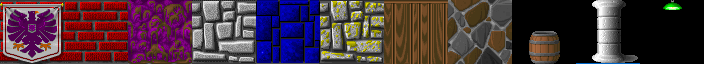The full code of the whole thing is given, it's similar to the code of Raycasting II, but with added code here and there.

The new variables for sprite casting are the struct Sprite, containing the position and texture of the sprite, the value numSprites: the number of sprites, the sprite array: defines the positions and textures of all the Sprites, the declaration of the bubbleSort function: this will sort the sprites, and the arrays used as arguments for this function: spriteOrder and spriteDistance, and finally, ZBuffer: this is the 1D equivalent of the ZBuffer in a true 3D engine. In the sprite array, every third number is the number of its texture, you can see which number means what there where the textures are loaded.

 ```#define screenWidth 640 #define screenHeight 480 #define texWidth 64 #define texHeight 64 #define mapWidth 24 #define mapHeight 24 int worldMap[mapWidth][mapHeight] = { {8,8,8,8,8,8,8,8,8,8,8,4,4,6,4,4,6,4,6,4,4,4,6,4}, {8,0,0,0,0,0,0,0,0,0,8,4,0,0,0,0,0,0,0,0,0,0,0,4}, {8,0,3,3,0,0,0,0,0,8,8,4,0,0,0,0,0,0,0,0,0,0,0,6}, {8,0,0,3,0,0,0,0,0,0,0,0,0,0,0,0,0,0,0,0,0,0,0,6}, {8,0,3,3,0,0,0,0,0,8,8,4,0,0,0,0,0,0,0,0,0,0,0,4}, {8,0,0,0,0,0,0,0,0,0,8,4,0,0,0,0,0,6,6,6,0,6,4,6}, {8,8,8,8,0,8,8,8,8,8,8,4,4,4,4,4,4,6,0,0,0,0,0,6}, {7,7,7,7,0,7,7,7,7,0,8,0,8,0,8,0,8,4,0,4,0,6,0,6}, {7,7,0,0,0,0,0,0,7,8,0,8,0,8,0,8,8,6,0,0,0,0,0,6}, {7,0,0,0,0,0,0,0,0,0,0,0,0,0,0,0,8,6,0,0,0,0,0,4}, {7,0,0,0,0,0,0,0,0,0,0,0,0,0,0,0,8,6,0,6,0,6,0,6}, {7,7,0,0,0,0,0,0,7,8,0,8,0,8,0,8,8,6,4,6,0,6,6,6}, {7,7,7,7,0,7,7,7,7,8,8,4,0,6,8,4,8,3,3,3,0,3,3,3}, {2,2,2,2,0,2,2,2,2,4,6,4,0,0,6,0,6,3,0,0,0,0,0,3}, {2,2,0,0,0,0,0,2,2,4,0,0,0,0,0,0,4,3,0,0,0,0,0,3}, {2,0,0,0,0,0,0,0,2,4,0,0,0,0,0,0,4,3,0,0,0,0,0,3}, {1,0,0,0,0,0,0,0,1,4,4,4,4,4,6,0,6,3,3,0,0,0,3,3}, {2,0,0,0,0,0,0,0,2,2,2,1,2,2,2,6,6,0,0,5,0,5,0,5}, {2,2,0,0,0,0,0,2,2,2,0,0,0,2,2,0,5,0,5,0,0,0,5,5}, {2,0,0,0,0,0,0,0,2,0,0,0,0,0,2,5,0,5,0,5,0,5,0,5}, {1,0,0,0,0,0,0,0,0,0,0,0,0,0,0,0,0,0,0,0,0,0,0,5}, {2,0,0,0,0,0,0,0,2,0,0,0,0,0,2,5,0,5,0,5,0,5,0,5}, {2,2,0,0,0,0,0,2,2,2,0,0,0,2,2,0,5,0,5,0,0,0,5,5}, {2,2,2,2,1,2,2,2,2,2,2,1,2,2,2,5,5,5,5,5,5,5,5,5} }; struct Sprite { double x; double y; int texture; }; #define numSprites 19 Sprite sprite[numSprites] = { {20.5, 11.5, 10}, //green light in front of playerstart //green lights in every room {18.5,4.5, 10}, {10.0,4.5, 10}, {10.0,12.5,10}, {3.5, 6.5, 10}, {3.5, 20.5,10}, {3.5, 14.5,10}, {14.5,20.5,10}, //row of pillars in front of wall: fisheye test {18.5, 10.5, 9}, {18.5, 11.5, 9}, {18.5, 12.5, 9}, //some barrels around the map {21.5, 1.5, 8}, {15.5, 1.5, 8}, {16.0, 1.8, 8}, {16.2, 1.2, 8}, {3.5, 2.5, 8}, {9.5, 15.5, 8}, {10.0, 15.1,8}, {10.5, 15.8,8}, }; Uint32 buffer[screenHeight][screenWidth]; // y-coordinate first because it works per scanline //1D Zbuffer double ZBuffer[screenWidth]; //arrays used to sort the sprites int spriteOrder[numSprites]; double spriteDistance[numSprites]; //function used to sort the sprites void sortSprites(int* order, double* dist, int amount); int main(int /*argc*/, char */*argv*/[]) { double posX = 22.0, posY = 11.5; //x and y start position double dirX = -1.0, dirY = 0.0; //initial direction vector double planeX = 0.0, planeY = 0.66; //the 2d raycaster version of camera plane double time = 0; //time of current frame double oldTime = 0; //time of previous frame std::vector texture; for(int i = 0; i < 11; i++) texture[i].resize(texWidth * texHeight);```

3 new textures are loaded: the sprites. There's nothing that stops you from loading more textures, or making the textures higher resolution.

 ``` screen(screenWidth, screenHeight, 0, "Raycaster"); //load some textures unsigned long tw, th, error = 0; error |= loadImage(texture, tw, th, "pics/eagle.png"); error |= loadImage(texture, tw, th, "pics/redbrick.png"); error |= loadImage(texture, tw, th, "pics/purplestone.png"); error |= loadImage(texture, tw, th, "pics/greystone.png"); error |= loadImage(texture, tw, th, "pics/bluestone.png"); error |= loadImage(texture, tw, th, "pics/mossy.png"); error |= loadImage(texture, tw, th, "pics/wood.png"); error |= loadImage(texture, tw, th, "pics/colorstone.png"); //load some sprite textures error |= loadImage(texture, tw, th, "pics/barrel.png"); error |= loadImage(texture, tw, th, "pics/pillar.png"); error |= loadImage(texture, tw, th, "pics/greenlight.png"); if(error) { std::cout << "error loading images" << std::endl; return 1; }```

Here's the main loop which starts with raycasting the floors and walls, same code as before.

 ``` //start the main loop while(!done()) { //FLOOR CASTING for(int y = 0; y < h; y++) { // rayDir for leftmost ray (x = 0) and rightmost ray (x = w) float rayDirX0 = dirX - planeX; float rayDirY0 = dirY - planeY; float rayDirX1 = dirX + planeX; float rayDirY1 = dirY + planeY; // Current y position compared to the center of the screen (the horizon) int p = y - screenHeight / 2; // Vertical position of the camera. float posZ = 0.5 * screenHeight; // Horizontal distance from the camera to the floor for the current row. // 0.5 is the z position exactly in the middle between floor and ceiling. float rowDistance = posZ / p; // calculate the real world step vector we have to add for each x (parallel to camera plane) // adding step by step avoids multiplications with a weight in the inner loop float floorStepX = rowDistance * (rayDirX1 - rayDirX0) / screenWidth; float floorStepY = rowDistance * (rayDirY1 - rayDirY0) / screenWidth; // real world coordinates of the leftmost column. This will be updated as we step to the right. float floorX = posX + rowDistance * rayDirX0; float floorY = posY + rowDistance * rayDirY0; for(int x = 0; x < screenWidth; ++x) { // the cell coord is simply got from the integer parts of floorX and floorY int cellX = (int)(floorX); int cellY = (int)(floorY); // get the texture coordinate from the fractional part int tx = (int)(texWidth * (floorX - cellX)) & (texWidth - 1); int ty = (int)(texHeight * (floorY - cellY)) & (texHeight - 1); floorX += floorStepX; floorY += floorStepY; // choose texture and draw the pixel int floorTexture = 3; int ceilingTexture = 6; Uint32 color; // floor color = texture[floorTexture][texWidth * ty + tx]; color = (color >> 1) & 8355711; // make a bit darker buffer[y][x] = color; //ceiling (symmetrical, at screenHeight - y - 1 instead of y) color = texture[ceilingTexture][texWidth * ty + tx]; color = (color >> 1) & 8355711; // make a bit darker buffer[screenHeight - y - 1][x] = color; } } // WALL CASTING for(int x = 0; x < w; x++) { //calculate ray position and direction double cameraX = 2 * x / double(w) - 1; //x-coordinate in camera space double rayDirX = dirX + planeX * cameraX; double rayDirY = dirY + planeY * cameraX; //which box of the map we're in int mapX = int(posX); int mapY = int(posY); //length of ray from current position to next x or y-side double sideDistX; double sideDistY; //length of ray from one x or y-side to next x or y-side double deltaDistX = (rayDirX == 0) ? 1e30 : std::abs(1 / rayDirX); double deltaDistY = (rayDirY == 0) ? 1e30 : std::abs(1 / rayDirY); double perpWallDist; //what direction to step in x or y-direction (either +1 or -1) int stepX; int stepY; int hit = 0; //was there a wall hit? int side; //was a NS or a EW wall hit? //calculate step and initial sideDist if (rayDirX < 0) { stepX = -1; sideDistX = (posX - mapX) * deltaDistX; } else { stepX = 1; sideDistX = (mapX + 1.0 - posX) * deltaDistX; } if (rayDirY < 0) { stepY = -1; sideDistY = (posY - mapY) * deltaDistY; } else { stepY = 1; sideDistY = (mapY + 1.0 - posY) * deltaDistY; } //perform DDA while (hit == 0) { //jump to next map square, either in x-direction, or in y-direction if (sideDistX < sideDistY) { sideDistX += deltaDistX; mapX += stepX; side = 0; } else { sideDistY += deltaDistY; mapY += stepY; side = 1; } //Check if ray has hit a wall if (worldMap[mapX][mapY] > 0) hit = 1; } //Calculate distance of perpendicular ray (Euclidean distance would give fisheye effect!) if(side == 0) perpWallDist = (sideDistX - deltaDistX); else perpWallDist = (sideDistY - deltaDistY); //Calculate height of line to draw on screen int lineHeight = (int)(h / perpWallDist); //calculate lowest and highest pixel to fill in current stripe int drawStart = -lineHeight / 2 + h / 2; if(drawStart < 0) drawStart = 0; int drawEnd = lineHeight / 2 + h / 2; if(drawEnd >= h) drawEnd = h - 1; //texturing calculations int texNum = worldMap[mapX][mapY] - 1; //1 subtracted from it so that texture 0 can be used! //calculate value of wallX double wallX; //where exactly the wall was hit if (side == 0) wallX = posY + perpWallDist * rayDirY; else wallX = posX + perpWallDist * rayDirX; wallX -= floor((wallX)); //x coordinate on the texture int texX = int(wallX * double(texWidth)); if(side == 0 && rayDirX > 0) texX = texWidth - texX - 1; if(side == 1 && rayDirY < 0) texX = texWidth - texX - 1; // How much to increase the texture coordinate per screen pixel double step = 1.0 * texHeight / lineHeight; // Starting texture coordinate double texPos = (drawStart - h / 2 + lineHeight / 2) * step; for(int y = drawStart; y> 1) & 8355711; buffer[y][x] = color; }```

After raycasting the wall, the ZBuffer has to be set. This ZBuffer is 1D, because it only contains the distance to the wall of every vertical stripe, instead of having this for every pixel. This also ends the loop through every vertical stripe, because rendering the sprites will be done outside this loop.

 ``` //SET THE ZBUFFER FOR THE SPRITE CASTING ZBuffer[x] = perpWallDist; //perpendicular distance is used } ```

After the floors and walls are finally drawn, the sprites can be drawn. This code is very unoptimized, a few improvements are explained later. First it sorts the sprites from far to close, so that the far ones will be drawn first. Then it projects each sprite, calculates the size it should have on screen, and draws it stripe by stripe. The matrix multiplication for the projection is very easy because it's only a 2x2 matrix. It comes in very handy again that they raycaster already used a 2D camera matrix, instead of representing the player with an angle and a position instead like some raycasters do.

The distance calculated for the sorting of the sprites is never used later on, because the perpendicular distance is used instead. For sorting the sprites it doesn't matter if you take the square root of the distance or not, so no calculation time is wasted for that.

 ``` //SPRITE CASTING //sort sprites from far to close for(int i = 0; i < numSprites; i++) { spriteOrder[i] = i; spriteDistance[i] = ((posX - sprite[i].x) * (posX - sprite[i].x) + (posY - sprite[i].y) * (posY - sprite[i].y)); //sqrt not taken, unneeded } sortSprites(spriteOrder, spriteDistance, numSprites); //after sorting the sprites, do the projection and draw them for(int i = 0; i < numSprites; i++) { //translate sprite position to relative to camera double spriteX = sprite[spriteOrder[i]].x - posX; double spriteY = sprite[spriteOrder[i]].y - posY; //transform sprite with the inverse camera matrix // [ planeX dirX ] -1 [ dirY -dirX ] // [ ] = 1/(planeX*dirY-dirX*planeY) * [ ] // [ planeY dirY ] [ -planeY planeX ] double invDet = 1.0 / (planeX * dirY - dirX * planeY); //required for correct matrix multiplication double transformX = invDet * (dirY * spriteX - dirX * spriteY); double transformY = invDet * (-planeY * spriteX + planeX * spriteY); //this is actually the depth inside the screen, that what Z is in 3D int spriteScreenX = int((w / 2) * (1 + transformX / transformY)); //calculate height of the sprite on screen int spriteHeight = abs(int(h / (transformY))); //using 'transformY' instead of the real distance prevents fisheye //calculate lowest and highest pixel to fill in current stripe int drawStartY = -spriteHeight / 2 + h / 2; if(drawStartY < 0) drawStartY = 0; int drawEndY = spriteHeight / 2 + h / 2; if(drawEndY >= h) drawEndY = h - 1; //calculate width of the sprite int spriteWidth = abs( int (h / (transformY))); int drawStartX = -spriteWidth / 2 + spriteScreenX; if(drawStartX < 0) drawStartX = 0; int drawEndX = spriteWidth / 2 + spriteScreenX; if(drawEndX >= w) drawEndX = w - 1; //loop through every vertical stripe of the sprite on screen for(int stripe = drawStartX; stripe < drawEndX; stripe++) { int texX = int(256 * (stripe - (-spriteWidth / 2 + spriteScreenX)) * texWidth / spriteWidth) / 256; //the conditions in the if are: //1) it's in front of camera plane so you don't see things behind you //2) it's on the screen (left) //3) it's on the screen (right) //4) ZBuffer, with perpendicular distance if(transformY > 0 && stripe > 0 && stripe < w && transformY < ZBuffer[stripe]) for(int y = drawStartY; y < drawEndY; y++) //for every pixel of the current stripe { int d = (y) * 256 - h * 128 + spriteHeight * 128; //256 and 128 factors to avoid floats int texY = ((d * texHeight) / spriteHeight) / 256; Uint32 color = texture[sprite[spriteOrder[i]].texture][texWidth * texY + texX]; //get current color from the texture if((color & 0x00FFFFFF) != 0) buffer[y][stripe] = color; //paint pixel if it isn't black, black is the invisible color } } }```

After it all is drawn, the screen is updated, and the input keys are handled.

 ``` drawBuffer(buffer); for(int y = 0; y < h; y++) for(int x = 0; x < w; x++) buffer[y][x] = 0; //clear the buffer instead of cls() //timing for input and FPS counter oldTime = time; time = getTicks(); double frameTime = (time - oldTime) / 1000.0; //frametime is the time this frame has taken, in seconds print(1.0 / frameTime); //FPS counter redraw(); //speed modifiers double moveSpeed = frameTime * 3.0; //the constant value is in squares/second double rotSpeed = frameTime * 2.0; //the constant value is in radians/second readKeys(); //move forward if no wall in front of you if (keyDown(SDLK_UP)) { if(worldMap[int(posX + dirX * moveSpeed)][int(posY)] == false) posX += dirX * moveSpeed; if(worldMap[int(posX)][int(posY + dirY * moveSpeed)] == false) posY += dirY * moveSpeed; } //move backwards if no wall behind you if (keyDown(SDLK_DOWN)) { if(worldMap[int(posX - dirX * moveSpeed)][int(posY)] == false) posX -= dirX * moveSpeed; if(worldMap[int(posX)][int(posY - dirY * moveSpeed)] == false) posY -= dirY * moveSpeed; } //rotate to the right if (keyDown(SDLK_RIGHT)) { //both camera direction and camera plane must be rotated double oldDirX = dirX; dirX = dirX * cos(-rotSpeed) - dirY * sin(-rotSpeed); dirY = oldDirX * sin(-rotSpeed) + dirY * cos(-rotSpeed); double oldPlaneX = planeX; planeX = planeX * cos(-rotSpeed) - planeY * sin(-rotSpeed); planeY = oldPlaneX * sin(-rotSpeed) + planeY * cos(-rotSpeed); } //rotate to the left if (keyDown(SDLK_LEFT)) { //both camera direction and camera plane must be rotated double oldDirX = dirX; dirX = dirX * cos(rotSpeed) - dirY * sin(rotSpeed); dirY = oldDirX * sin(rotSpeed) + dirY * cos(rotSpeed); double oldPlaneX = planeX; planeX = planeX * cos(rotSpeed) - planeY * sin(rotSpeed); planeY = oldPlaneX * sin(rotSpeed) + planeY * cos(rotSpeed); } } }```

The sortSprites sorts the sprites from farthest away to closest by distance. It uses the standard std::sort function provided by C++. But since we need to sort two arrays using the same order here (order and dist), most of the code is spent moving the data into and out of a vector of pairs.

 ```//sort algorithm //sort the sprites based on distance void sortSprites(int* order, double* dist, int amount) { std::vector> sprites(amount); for(int i = 0; i < amount; i++) { sprites[i].first = dist[i]; sprites[i].second = order[i]; } std::sort(sprites.begin(), sprites.end()); // restore in reverse order to go from farthest to nearest for(int i = 0; i < amount; i++) { dist[i] = sprites[amount - i - 1].first; order[i] = sprites[amount - i - 1].second; } } }```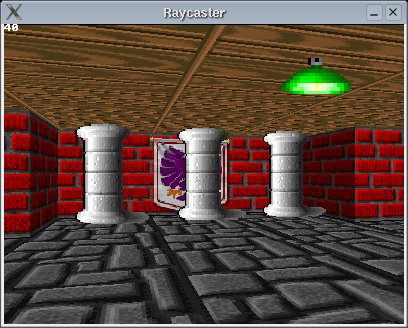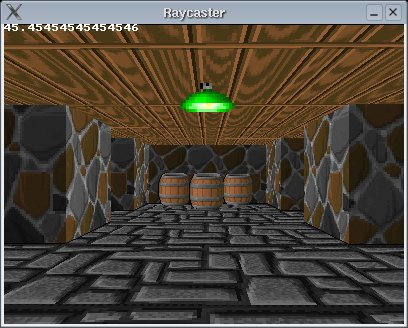The green light is a very small sprite, but the program still goes through all its invisible pixels to check their color. It could be made faster by telling which sprites have large invisible parts, and only drawing a smaller rectangular part of them containing all visible pixels.

To make some objects unwalkthroughable, you can either check the distance of the player to every object when he moves for collision detection, or, make another 2D map that contains for every square if it's walkthroughable or not, this can be used for walls as well.

In for example Wolfenstein 3D, some objects (for example the soldiers) have 8 different pictures when viewing it from different angles, to make it appear as if the sprite is really 3D. You can get the angle of the object to the player for example with the atan2 function, and then choose 1 of 8 textures depending on the angle. You can also give the sprites even more textures for animation.

## Scaling Sprites

It's pretty easy to let the program draw the sprites larger or smaller, and move the sprites up or down. To shrink the sprite, divide spriteWidth and spriteHeight through something. If you halve the height of the sprites, for example the pillar, then the bottom will move up so that the pillar appears to be floating. That's why in the code below, apart from the parameters uDiv and vDiv to shrink the sprite, also a parameter vMove is added to move the sprite down if it has to stand on the floor, or up if it has to hang on the ceiling. vMoveScreen is vMove projected on the screen by dividing it through the depth.

 ``` //parameters for scaling and moving the sprites #define uDiv 1 #define vDiv 1 #define vMove 0.0 int vMoveScreen = int(vMove / transformY); //calculate height of the sprite on screen int spriteHeight = abs(int(h / (transformY))) / vDiv; //using 'transformY' instead of the real distance prevents fisheye //calculate lowest and highest pixel to fill in current stripe int drawStartY = -spriteHeight / 2 + h / 2 + vMoveScreen; if(drawStartY < 0) drawStartY = 0; int drawEndY = spriteHeight / 2 + h / 2 + vMoveScreen; if(drawEndY >= h) drawEndY = h - 1; //calculate width of the sprite int spriteWidth = abs( int (h / (transformY))) / uDiv; int drawStartX = -spriteWidth / 2 + spriteScreenX; if(drawStartX < 0) drawStartX = 0; int drawEndX = spriteWidth / 2 + spriteScreenX; if(drawEndX >= w) drawEndX = w - 1; //loop through every vertical stripe of the sprite on screen for(int stripe = drawStartX; stripe < drawEndX; stripe++) { int texX = int(256 * (stripe - (-spriteWidth / 2 + spriteScreenX)) * texWidth / spriteWidth) / 256; //the conditions in the if are: //1) it's in front of camera plane so you don't see things behind you //2) it's on the screen (left) //3) it's on the screen (right) //4) ZBuffer, with perpendicular distance if(transformY > 0 && stripe > 0 && stripe < w && transformY < ZBuffer[stripe]) for(int y = drawStartY; y < drawEndY; y++) //for every pixel of the current stripe { int d = (y-vMoveScreen) * 256 - h * 128 + spriteHeight * 128; //256 and 128 factors to avoid floats int texY = ((d * texHeight) / spriteHeight) / 256; Uint32 color = texture[sprite[spriteOrder[i]].texture][texWidth * texY + texX]; //get current color from the texture if((color & 0x00FFFFFF) != 0) buffer[y][stripe] = color; //paint pixel if it isn't black, black is the invisible color } } }```

When uDiv = 2, vDiv = 2, vMove = 0.0, the sprites are half as big, and float: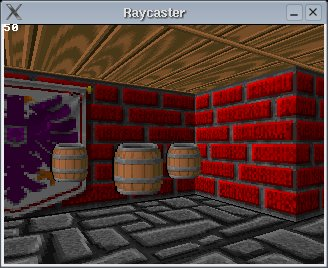Put it back on the ground by setting vMove to 64.0 (the size of the texture):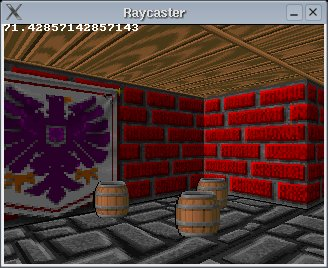If you make vMove even higher to place the sprites under the ground, they'll still be drawn through the ground, because the ZBuffer is 1D and can only detect if the sprite is in front or behind a wall.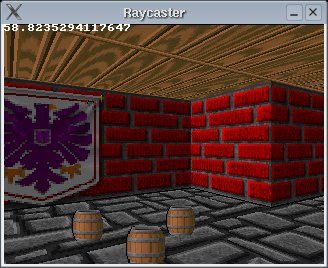Of course, by lowering the barrels, the green light is lower too so it doesn't hang on the ceiling anymore. To make this useful, you have to give each sprite its own uDiv, vDiv and vMove parameters, for example you can put them in the sprite struct.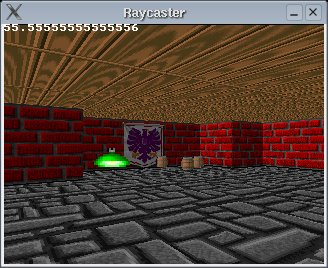## Translucent Sprites

Because we're working in RGB color, making sprites translucent is very simple. All you have to do is take the average of the old color in the buffer and the new color of the sprite. Old games like for example Wolfenstein 3D used a palette of 256 colors with no logical mathematical rules for the colors in the palette, so there translucency wasn't so easy. Change the following line of code

 `if((color & 0x00FFFFFF) != 0) buffer[y][stripe] = color; //paint pixel if it isn't black, black is the invisible color`

into

 `if((color & 0x00FFFFFF) != 0) buffer[y][stripe] = RGBtoINT(INTtoRGB(buffer[y][stripe]) / 2 + INTtoRGB(color) / 2); //paint pixel if it isn't black, black is the invisible color`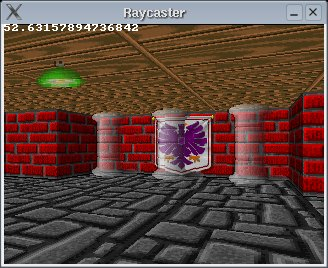To make them more translucent, try something like

 `if((color & 0x00FFFFFF) != 0) buffer[y][stripe] = RGBtoINT(3 * INTtoRGB(buffer[y][stripe]) / 4 + INTtoRGB(color) / 4); //paint pixel if it isn't black, black is the invisible color`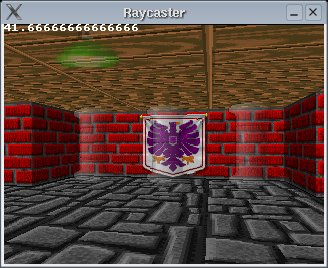You can also try more special tricks, for example translucent sprites, that make the walls behind them of negative color:

 `if((color & 0x00FFFFFF) != 0) buffer[y][stripe] = RGBtoINT((RGB_White - INTtoRGB(buffer[y][stripe])) / 2 + INTtoRGB(color) / 2); //paint pixel if it isn't black, black is the invisible color`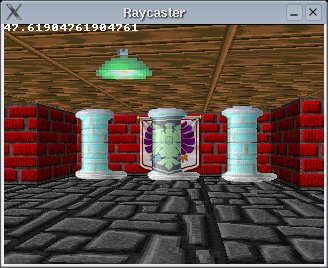To be useful for a game, it would be more handy to give each sprite its own translucency effect (if any), with an extra parameter in the sprite struct. For example the green light could be translucent, but a pillar certainly not.

Last edited: 2020